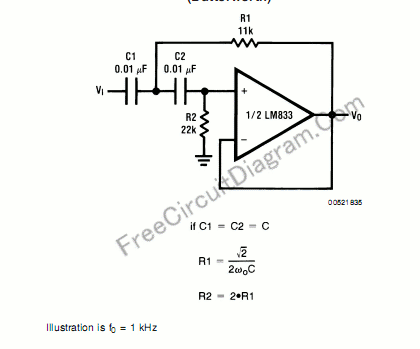# Butterworth Second Order High-Pass Filter: The Circuit and The FormulaFor high pass filter, this circuit is similar to low-pass filter circuit, but the position for resistors and capacitor are interchanged. Here is the circuit’s schematic and formula:Similar with low pass design guide, the resistor and capacitor should be chosen according to the formula, and the resistor value should be:

• Much higher than equivalent leakage resistance of the capacitor.
• Much higher than the operational-amplifier’s (op-amp’s) input impedance.
• Doesn’t draw excessive current-violating the maximum allowed op-amp’s output current.

In general, for higher capacitor value, it’s leakage current would be higher and you must use lower resistors to compensate the capacitor’s current leakage. [Circuit’s schematic source: National Semiconductor’s LM833 Application Notes]